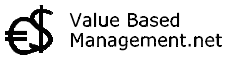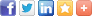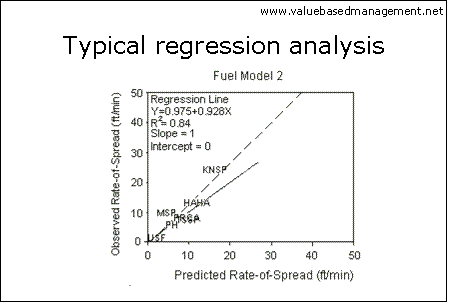## Statistical ForecastingCategories: Articles  |  Books  |  Dictionary  |  Faq  |  Home

# Regression Analysis

Summary of Regression Analysis. AbstractRegression Analysis can predict the outcome of a given key business indicator (dependent variable) based on the interactions of other related business drivers (explanatory variables). For example you could predict sales volume based on the the amount spent on advertising and the number of sales people you employ. Of course, a real model would need more variables and is much more complex. Although nobody can really look into the future, modern statistical methods, econometric models and business intelligence software go a long way in helping businesses forecast and estimate what is going to happen in the future.   Regression analysis is a Statistical Forecasting model that is concerned with describing and evaluating the relationship between a given variable (usually called the dependent variable) and one or more other variables (usually known as the independent variables. Regression analysis models are used to help us predict the value of one variable from one or more other variables whose values can be predetermined. The first stage of the process is to identify the variable we want to predict (the dependent variable) and to then carry out multiple regression analysis focusing on the variables we want to use as predictors (explanatory variables). The multiple regression analysis would then identify the relationship between the dependent variable and the explanatory variables – this is then finally presented as a model (formula). Compare also: Dynamic Regression  |  Exponential Smoothing  |  ARIMA  |  Operations Research
 ©2016 Value Based Management.net - Last updated: Jan 6th, 2016 - All names ™ by their owners